output.to from Sideway

Draft for Information Only

# Content

` Acoustic Plane Wave Properties  Acoustic Pressure  Acoustic Pressure Addition`

## Acoustic Plane Wave Properties

The acoustic pressure caused by an acoustic disturbance varies with both time and position. Besides studying of the fluctuation of acoustic pressure that associated with a sound wave with respective to time at any point, many practical problems focus on the pressure variation caused by the sound at any point with respective to position.

### Acoustic Pressure

The acoustic pressure at any point varies with time sinusoidally, the root mean square is more convenient to be used to measure the alternating pressure fluctuation by taking the square root of the average of squares of instantaneous acoustic pressures within a period T. That is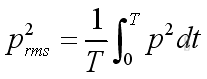where

prms is the root mean square of acoustic pressures
T is the period of measurement
p is the instantaneous acoustic pressures

The instantaneous acoustic pressure can be expressed as: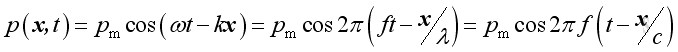where

p(x,t) is the instantaneous acoustic pressures
x is distance
t is time
pm is the maximum of the instantaneous acoustic pressures
ω is angular velocity
k is wave number
ƒ is frequency
λ is wave length

Therefore: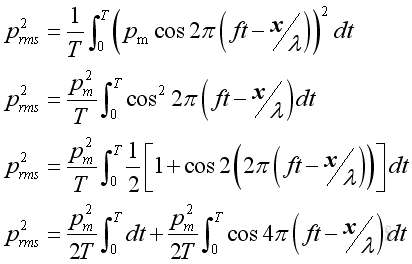Since the time average of a cosine function of function of time equals to zero, imply :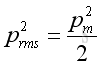or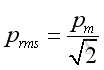### Acoustic Pressure Addition

The addition of two sound pressures of different frequency equals to: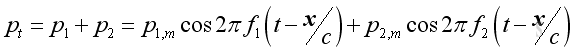Imply the square of the total sound pressure is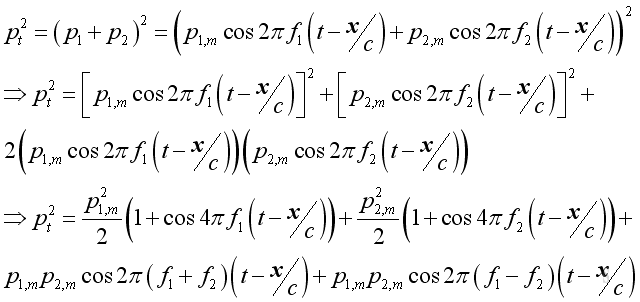The root mean square value of the total the total acoustic pressure can be obtained by taking the time average process. Similarly, the cosine term of function of time equal s to zero, and get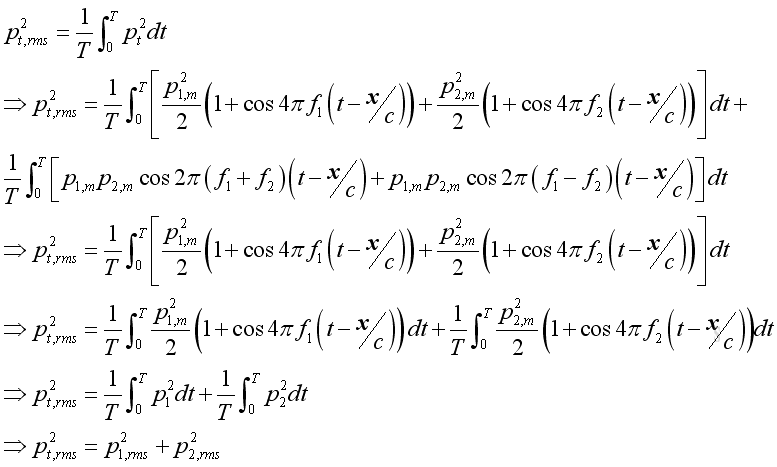Therefore. the total acoustic pressure of the two equal sound pressure due to two noises of different frequencies are only the √2 times of the sound pressure due to either noise source. The result can also be extended to a continuous bands of frequencies. However, when the two frequencies are equal and coherent, the result will be different.

When there is the present of phase angle, the result becomes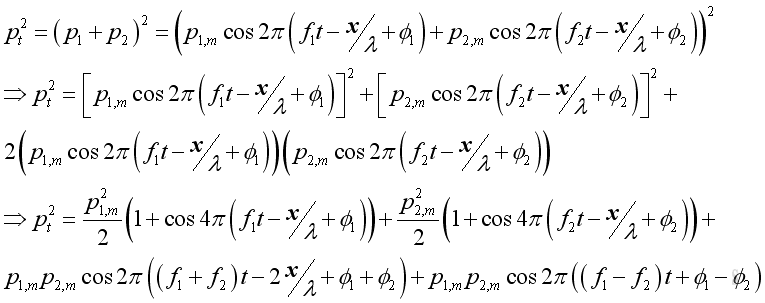Since the time average of the cosine terms of functions of time is zero, the phase angle does not affect the result of sounds with different frequencies. However, the phase angle affects the result when the frequencies of two sound sources are the same. By taking the time average, the equation with same frequency becomes: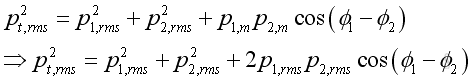Acoustic Intensity

Acoustic intensity is the sound power passing through a unit area, it can be considered as the rate of transmitting energy through the medium cross section area by medium fluctuation due to sound wave. The vibration of medium composes the change in both potential and kinetic energy.

Assuming constant mass and at initial state both potential energy and kinetic energy are zero with the acoustic disturbance equals zero. The potential energy EPE and kinetic energy EKE of an infinitesimal element of volume Vo due to acoustic fluctuation along x direction is: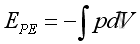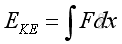From the linearized continuity equation, where s is the acoustic displacement,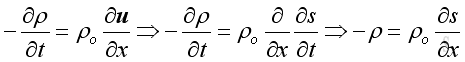Substitute the speed of propagation,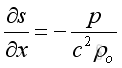The volume of the infinitesimal element can be expressed as,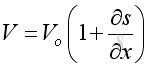Substitute the differential in term of pressure, then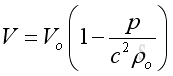The differential volume can then be equals to: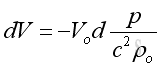Therefore the potential energy is: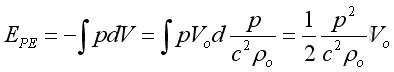For the kinetic energy, substitute the velocity in term of pressure :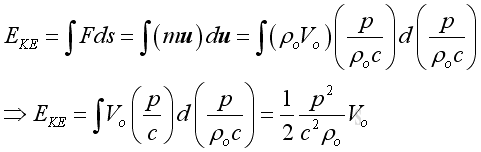Therefore the total instantaneous energy of an infinitesimal element due to acoustic disturbance is: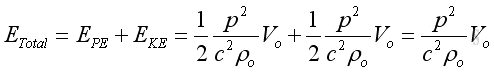Since the wave propagate at the speed of s, the volume of infinitesimal element per unit area in time δt  can be expressed as: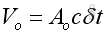As the instantaneous intensity is the rate of energy flow through a unit area, imply: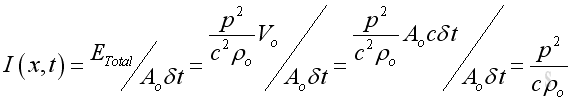Or, in term of acoustic pressure and acoustic velocity: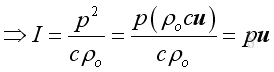Similar to the pressure, the intensity usually expressed as an average in practice, imply: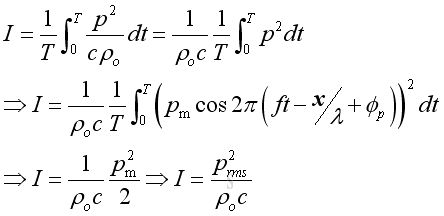In form of complex exponential representation, the intensity equals to the real active component of intensity: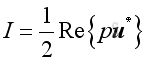Imply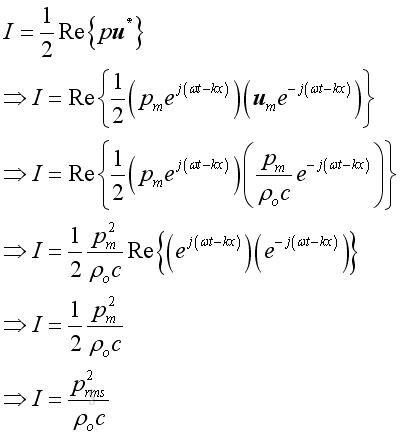Acoustic Power of Plane Wave

Sound power is defined as the integration of intensity over the surface area enclosing the enclosure and is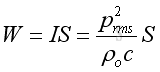SPL and SIL of Plane Wave

The Sound Pressure Level of a plane wave: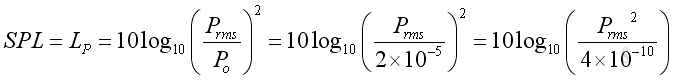Assume ρo = 1.2kg/m3, c = 334m/s,the Sound Intensity Level of a plane wave: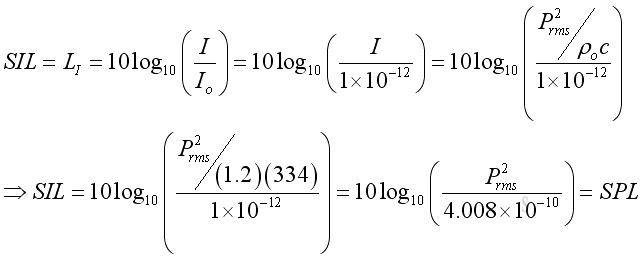Plane Wave Example

A plane wave propagates with a frequency of 50Hz and maximum pressure amplitude 150Pa in positive x direction with characteristic that at time t = 0 and at x = 0, the plane wave in term of acoustic pressure can be expressed as: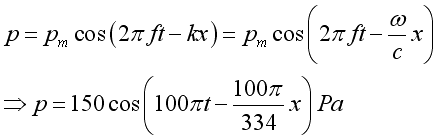The corresponding plane wave in term of medium velocity can be expressed as: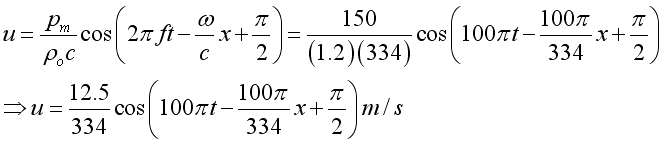The mean intensity of the plane wave can be expressed as: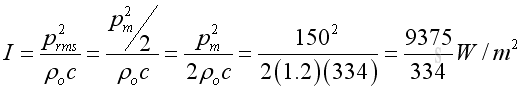The sound intensity level of the plane wave in term of dB can be expressed as: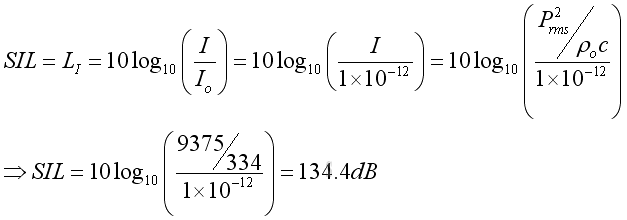©sidewayReferences

1. Michael P. Norton; Denis G. Karczub,, 2003, Fundamentals of Noise and Vibration Analysis for Engieer, Cambridge, United Kingdom
2. G. Porges, 1977, Applied Acoustics, Edward Arnold Limited, Britain
3. Douglas D. Reynolds, 1981, Engineering Principles of Acoustics:; Noise and Vibration Control, Allyn and Bacon, USA
4. Conrad J. Hemond, 1983, Engineering Acoustics & Noise Control, Prentice-Hall, USA
5. F. Fahy, 2001, Foundations of Engineering Acoustics, Academic Press, UK
6. D.A. Biew; C.H. Hansen, 1996, Engineering Noise Control: Theory and Practice, E & FN Spon, New YorkID: 100900023 Last Updated: 10/9/2010 Revision: 1 Ref:Home (5)

Business

Management

HBR (3)

Information

Recreation

Hobbies (7)

Culture

Chinese (1097)

English (336)

Reference (66)

Computer

Hardware (149)

Software

Application (187)

Digitization (24)

Numeric (19)

Programming

Web (644)CSS (SC)

ASP.NET (SC)

HTML

Knowledge Base

Common Color (SC)

Html 401 Special (SC)

OS (389)MS Windows

Windows10 (SC)

.NET Framework (SC)

DeskTop (7)

Knowledge

Mathematics

Formulas (8)

Number Theory (206)

Algebra (20)

Trigonometry (18)

Geometry (18)

Calculus (67)

Complex Analysis (21)

Engineering

Tables (8)

Mechanical

Mechanics (1)

Rigid Bodies

Statics (92)

Dynamics (37)

Fluid (5)

Control

Acoustics (19)

Biology (1)

Geography (1)

Latest Updated Links

Copyright © 2000-2019 Sideway . All rights reserved Disclaimers last modified on 10 Feb 2019